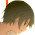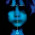## Friday, May 25, 2018

### A prefix xor week

The Apr 30 - May 6 week was about early qualification rounds for the major tournaments. TopCoder Open 2018 Round 1B took place on Thursday (problems, results, top 5 on the left, parallel round results, analysis). The medium problem provided ample challenge opportunities, and Michael_Levin has capitalized on those and earned the top spot with quite some margin — congratulations!

In the parallel round, kotamanegi was able to find even more challenges, and thus earned the top spot in the all-time high score list — congratulations as well :)

Google Code Jam 2018 Round 1C followed on Saturday (problems, results, top 5 on the left, analysis). The somewhat unusual interactive second problem did not delay Eryx much, as he finished the round in just over 35 minutes (8 seconds faster than austrin in 3rd place). Well done!

In my previous summary, I have mentioned a cute AtCoder problem: you are given a tree with 200000 vertices, each containing a number, either 0 or 1. We need to put all its vertices in some order in such a way that each vertex except the root appears to the right of its parent. We will then write out a sequence of 0s and 1s corresponding to numbers in the vertices in this order. What is the minimum possible number of inversions in this sequence? An inversion is a pair of positions such that the number in the left position is 1, and the number in the right position is 0.

The key idea that is widely applicable in this type of problems is called the exchange argument. Suppose we have some way to put the vertices in order, which results in some sequences of 1s and 0s. Let's consider two consecutive blocks of numbers in the resulting sequence, for example one block contains a 1s and b 0s, and the following block contains c 1s and d 0s. What happens to the number of inversions if we swap those blocks? It will change by cb-ad. Thus in an optimal sequence for every pair of consecutive blocks that are swappable (with respect to the parent-child constraint) we must have cb-ad>=0, which we can rewrite as c/d-a/b>=0.

Now suppose we have identified the optimal sequence for all children of some vertex, and want to find the optimal sequence for the vertex itself. The number for this vertex needs to go first, and then we need to interleave the sequences for the children somehow. In theory we could also reorder the optimal sequences for the children, but intuitively it is not necessary, and it will become clearer after we complete the solution.

Interleaving the sequences for the children can be viewed as splitting each child sequence into blocks, and then interleaving those blocks. From the exchange argument above, we need to take the block with the lowest fraction of 1s every time (lowest value of a/b).

There are many ways to split each child sequence into blocks, but we can notice that the first block must be at least the prefix p with the lowest fraction of 1s: in case we take a smaller prefix, the remaining part of p has an even lower fraction of 1s, and thus by the exchange argument above we'll be able to drag it to the beginning and improve the solution.

By applying the same argument repeatedly, we arrive at the following solution: each child sequence is split into blocks by taking the prefix with the lowest fraction of 1s as the first block, then the prefix with the lowest fraction of 1s of the remaining sequence as the second block, and so on. It's not hard to see that the fractions of 1s in those blocks for one child sequence will be nondecreasing, so in order to interleave those blocks we just sort them by the fraction, and blocks within one sequence will keep the same order.

It is however too slow to split each child sequence into blocks for every vertex, as potentially each sequence has a linear number of blocks, so the overall algorithm would be quadratic. However, since we interleave the blocks in nondecreasing order of fraction, we can notice that the sequence of blocks for each vertex is obtained by interleaving the sequences for the children — we don't need to re-split it. Then we prepend either a 0 or a 1 in the beginning. If it's a 0, we can simply make it a separate block and we'll still get a nondecreasing sequence. If it's a 1, it will "eat" a few following blocks until our sequence becomes nondecreasing.

This leads to the following approach: for each vertex, we'll build a sequence of blocks, ordered by the fraction of 1s, stored as a balanced search tree or a priority queue. In order to build the sequence for a vertex, we need to merge the sequences from its children, and add a new block in the beginning corresponding to the value in the vertex, and then repeatedly merge that block with the next block while its fraction is higher. When merging, we apply another relatively standard trick and insert blocks from the smaller sequence into the larger sequence, which guarantees that each block would be inserted at most log(n) times, and thus overall running time would be O(n*log2(n)).

I derive additional pleasure from the fact that while building this solution, we have essentially understood the mechanics of the problem in great detail: while initially we had a great deal of freedom in writing out the sequence, and it was not clear why we can even find the optimal sequence in polynomial time, now we realize that we just interleave blocks and merge them.

I have also mentioned a Codeforces problem: you are given 100000 numbers ai, each up to 260. You need to put them in such order that the xors of all prefixes of the resulting sequence form a strictly increasing sequence themselves.

The solution to this problem is quite the opposite to the previous one: instead of building a detailed understanding, we come up with an argument that works somewhat magically. It is not hard to prove that the solution works, but it keeps looking unexpected and magical.

Let's look at the highest bit set in at least one of ai's. Suppose it is set in some subset of numbers. Then the prefix xors will have 0 in this bit before the first such number, 1 in this bit after the first such number but before the second such number, 0 in this bit after the second such number, and so on. Since the resulting sequence must be non-decreasing, we must actually have exactly one such number. Let's start forming our resulting sequence by putting just this number there. Now we have two parts of the sequence, before this number and after it (including the number itself), which are somewhat independent: no matter which numbers go to the before part, its xor has 0 in the highest bit, and after xoring in our number the highest bit becomes 1, so we're guaranteed to have a strict increase on the boundary of the parts.

Now let's look at the next highest bit set in at least one of the remaining ai's, and look at the numbers where this bit is set. Just as before, the prefix xors will have 0 in this bit before the first such number, 1 in this bit after the first such number but before the second such number, 0 in this bit after the second such number, and so on. The only difference is that the set of numbers with this bit set might include the number that we already have in the sequence. If this is the case, then even though our bit will change from 1 to 0, we'd still have an increasing sequence, since a higher bit will change from 0 to 1 at the same point. So in case the already placed number has 1 in our bit, we can add two numbers with our bit as the highest bit: one in the beginning, and one after the already placed number. And in case the already placed number has 0 in our bit, we can add at most one number with our bit as the highest bit. Once again, after doing that, our sequence is guaranteed to be increasing at the boundaries of already placed numbers no matter what we insert between them.

A general step of our algorithm looks like this: we already have all numbers with highest bit higher than x placed, and want to place the numbers where the highest bit is x. We insert one such number in the beginning, and one after each already placed number where bit x is set, going from left to right, until we have no more numbers where the highest bit is x. If we run out of places before we run out of such numbers, there's no solution.

Note that the solution works just barely: for example, we can't put the new numbers after any subset of already placed numbers where bit x is set: we must go from left to right instead, to guarantee that the prefix xor does not have bit x set at each insertion point.  That's why I can't get rid of the feeling that this solution works almost by accident.

Thanks for reading, and check back for more!

1.It seems these pictures are the summer palace in peking. lol

2.Thanks Petr for sharing this. I find that a lot of the concepts are descriptive which I often find hard to understand. If you could include a couple of examples to illustrate the concept, it would be much more easier to understand.

3.please include some example in your explanation .
nice eplanation thank you

4.Wonderful Content. Thank you.